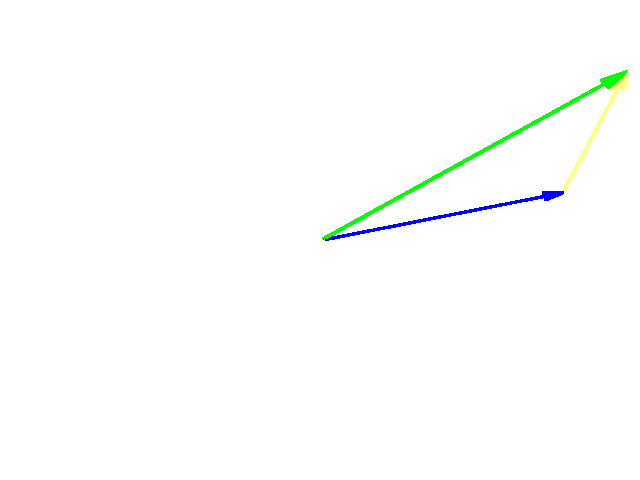September 8, 2016

# The Circle Tutorial

The sum of two vectors is itself a vector. It is calculated by adding the similar elements of the two vectors (i.e. Xsum=X1+X2, Ysum=Y1+Y2 and Zsum=Z1+Z2) but this is handled for you by the API function mathVecAdd(float *c, float *a, float *b, int n). The parameters are the result vector, the first vector to add, the second vector to add and finally an integer representing how many elements each vector has (i.e. 3.) Remember that in general vectors can have any number of dimensions or elements, so most vector functions take this last parameter that indicates the number of dimensions. Subtracting vectors is done much the same way although each resulting element is the difference of the two elements or by using mathVecSubtract(). (mathVecAdd performs c=a+b and mathVecSubtract performs c=a-b.)

Consider the following example:As any graduate of finger painting 101 knows… blue + yellow = green and what is easier to do with vectors than with finger paint is that green – yellow = blue.

So now we can add and subtract vectors, so what? 3D navigation involves a lot of vector manipulation that can be quite powerful. For instance, let’s replicate the old story about having a donkey follow a carrot on a stick. In this story a donkey follows a carrot tied to a stick that is always just out of reach. If you recall our state information includes a vector for our orientation – the direction in which we are “facing”. That vector is a unit vector that points in the direction we’re facing, but it does not point to a point in front of us, it points to a point one unit from the origin in the same direction we’re facing. If we use setPositionTarget and passed that vector we would simply travel to that point in space and stop, not what we’re looking for. That orientation vector points in the direction we’re facing, but to find the position that is “that-a-way” from “here,” we need to add the “that-a-way” vector to the “here” vector or add our orientation vector to our position vector. The result will be a position one unit ahead of our current position. If we keep trying to go to a position one unit ahead of us over and over, we will replicate the donkey and the carrot. Re-write your loop() function as the following:

``````void loop(){
DEBUG(("\nHello Space! Time: %d",time));
float Carrot;
float MyState;

api.getMyZRState(MyState);